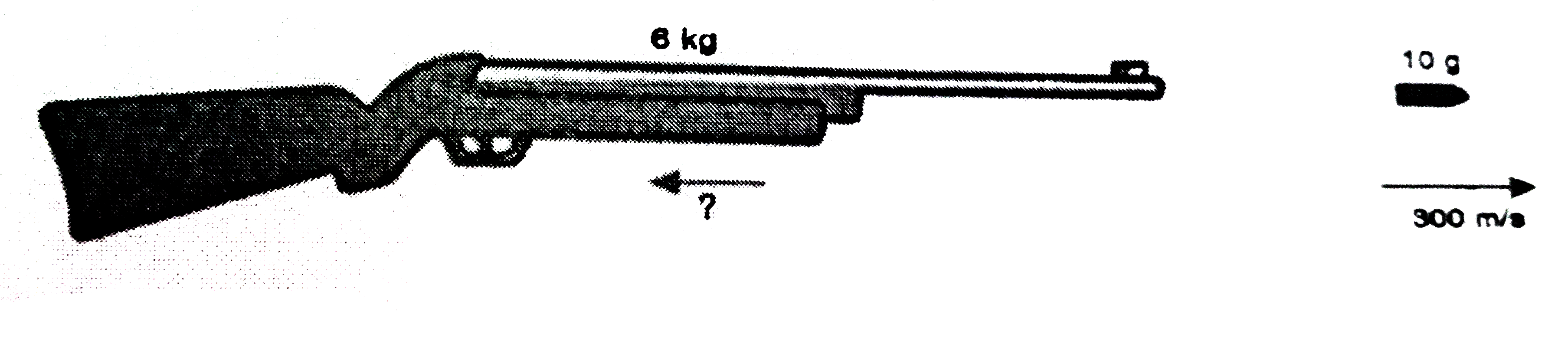Question

Updated on:30/05/2023

# A bullet of mass 10 g is fired from a gun of mass 6 kg with a velocity of 300 m/s. Calculate the recoil velocity of the gun.Text Solution

A
0.5 m/s
B
1 m/s
C
10 m/s
D
5 m/s
Solution
Here, Mass of bullet=10 g
=10100 kg
=0.01 kg
Velocity of bullet=300 m/s
Mass of gun=6kg
And, Recoil velocity of gun=? (To be calculated)
Now, putting these values in the relation :
Mass of bullet ×Velocity of bullet×=mass of gun ×Recoil velocity of gun
We get : 0.01×300=6× Recoil velocity of gun
So, Recoil velocity of gun=0.01×3006
=0.5 m/s
Note. The above prblem can also be solved by calculating the momentum of bullet and the gun separately as follows :
Momentum of bullet=Mass of bullet×Velocity of bullet
=0.01×300
= 3 kg. m/s
Now, suppose the recoil velocity of gun is v m/s.
So, Momentum of gun=Mass×Recoil velocity of gun
=6×v kg m/s
According to the law of conservation of momentum :
So, 3=6×v
And, v=36m/s
Recoil velocity of gun,v=0.5 m/s